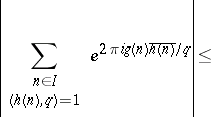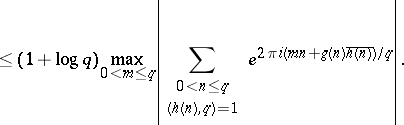# Exponential sum estimates

Exponential sums have the form

\begin{equation*} S = \sum _ { n \in A } e ^ { 2 \pi i f ( n ) }, \end{equation*}

where $A$ is a finite set of integers and $f$ is a real-valued function (cf. also Trigonometric sum). The basic problem is to show, under suitable circumstances, that $S = o ( \# A )$ as $\# A \rightarrow \infty$. Unless there are obvious reasons to the contrary one actually expects $S$ to have order around $( \# A ) ^ { 1 / 2 }$. Exponential sums in more than one variable also occur, and much of what is stated below can be generalized to such sums.

## Arithmetic sums.

There are two common types of exponential sum encountered in analytic number theory. In the first type, one starts with polynomials $g ( X ) , h ( X ) \in \mathbf{Z} [ X ]$, a positive integer modulus $q$, and a finite interval $I \subset \mathbf{R}$. One then takes $A$ as the set of integers $n \in I$ for which $\operatorname{GCD} ( h ( n ) , q ) = 1$, and sets $f ( n ) = g ( n ) \overline { h ( n ) } / q$, where $\overline { h ( n ) }$ is any integer for which $h ( n ) \overline { h ( n ) } \equiv 1 ( \operatorname { mod } q )$. When $I = ( 0 , q ]$, such a sum is called complete. When $I \subseteq ( 0 , q ]$ one may estimate the incomplete sum in terms of complete ones via the boundComplete sums have multiplicative properties which enable one to reduce consideration to the case in which $q$ is a prime number or a power of a prime number. Moreover, Weil's Riemann hypothesis for curves over finite fields (see [a4], for example) shows that, if $q$ is prime, then

\begin{equation*} \left| \sum _ { n \in I \atop ( h ( n ) , q ) = 1 } e ^ { 2 \pi i g ( n ) \overline { h ( n )} / q } \right| \leq ( \operatorname { deg } ( g ) + \operatorname { deg } ( h ) ) \sqrt { q }, \end{equation*}

except when $g ( n ) \overline { h ( n ) }$ is constant modulo $q$. For powers of a prime number the situation is more complicated, but broadly similar. It follows that, for fixed $g$ and $h$ and $q$ prime, the incomplete sum will be $o ( \# A )$ as soon as $\# A / ( \sqrt { q }\operatorname { log } q ) \rightarrow \infty$. It is an important outstanding problem (as of 2000) to improve on this in general.

## Analytic sums.

The second type of exponential sum arises when $f$ extends to a suitably smooth function on a real interval $I$. Important examples correspond to $f ( n ) = \alpha n ^ { k }$ for $k \in \mathbf{N}$, or $f ( n ) = ( t / 2 \pi ) \operatorname { log } n$, which occur in the theory of Waring's problem and the Riemann zeta-function, respectively (cf. also Waring problem; Zeta-function). Let $I = ( N , N + M ]$ with positive integers $M \leq N$. One typically imposes the condition that $f \in C ^ { \infty } [ N , N + M ]$ with

\begin{equation} \tag{a1} c _ { k } T N ^ { - k } \leq \left| f ^ { ( k ) } ( x ) \right| \leq c _ { k } ^ { \prime } T N ^ { - k } \end{equation}

\begin{equation*} ( k \in {\bf N} , N \leq x \leq N + M ), \end{equation*}

for suitable constants, ${ c } _ { k } ^ { \prime }$. Methods due to H. Weyl (see [a5], Chap. 2), J. van der Corput (see [a1]), I.M. Vinogradov and N.M. Korobov (see [a3], Chap. 6), and E. Bombieri and H. Iwaniec (see [a2]) have been used for sums of this type.

### Van der Corput's method.

Of the above approaches, the method of van der Corput is perhaps the most versatile. It is based on two processes, which convert the original sum $S$ into other sums. The A-process uses the inequality

\begin{equation*} \left| \sum _ { M < n \leq M + N } e ^ { 2 \pi i f ( n ) } \right| ^ { 2 } \ll \end{equation*}

\begin{equation*} \ll \frac { N ^ { 2 } } { H } + \frac { N } { H } \sum _ { 1 \leq h \leq H } \left| \sum_ { M < n \leq M + N - h } e ^ { 2 \pi i ( \, f ( n + h ) - f ( n ) ) } \right|, \end{equation*}

where $H \in \mathbf{N}$ satisfies $H \leq N$. This has the effect of replacing $f$ by a function $f ( x + h ) - f ( x )$ which satisfies (a1) with a smaller value of $T$. To describe the B-process it is assumed that $f ^ { \prime }$ is decreasing, and one writes $f ^ { \prime } ( M + N ) = A$, $f ^ { \prime } ( N ) = B$ and $f ^ { \prime } ( x _ { m } ) = m$ for $A < m \leq A + B$. The B-process then derives from the estimate

\begin{equation*} \sum _ { M < n \leq M + N } e ^ { 2 \pi i f ( n ) } = \end{equation*}

\begin{equation*} = e ^ { - i \pi / 4 } \sum _ { A < m \leq A + B } | f ^ { \prime } ( x _ { m } ) | ^ { - 1 / 2 } e ^ { 2 \pi i ( f ( x _ { m } ) - m x _ { m } ) } + \end{equation*}

\begin{equation*} + O ( T ^ { 1 / 3 } ) + O ( N ^ { 2 } T ^ { - 1 / 2 } ), \end{equation*}

which may be viewed as a form of the Poisson summation formula. When $T \ll N ^ { 2 }$, the B-process transforms $S$ into a sum with the same value of $T$ but a shorter range. In applications one uses the A-process repeatedly, with various values of $H$, until one has a sum to which the B-process can be applied effectively. One may then estimate the resulting shorter sum either via a trivial bound or by repeating the argument. In particular, $k - 2$ iterations of the A-process, followed by the B-process and a trivial estimate lead to van der Corput's $k$th derivative estimate: Let $k \geq 2$ be an integer, and let $f \in C ^ { k } [ N , N + M ]$ with $M$, $N$ integers. Suppose that $0 < \lambda _ { k } \leq | f ^ { ( k ) } ( x ) | \leq A \lambda _ { k }$ on $[ N , N + M ]$. Then, if $K = 2 ^ { k - 1 }$, one has

\begin{equation*} \sum _ { M < n \leq M + N } e ^ { 2 \pi i f ( n ) } \ll \end{equation*}

\begin{equation*} \ll A ^ { 2 / K } N \lambda _ { k } ^ { 1 / ( 2 K - 2 ) } + M ^ { 1 - 2 / K } \lambda _ { k } ^ { - 1 / ( 2 K - 2 ) }, \end{equation*}

uniformly in $k$.

### Exponent pairs.

Many bounds of the form $S \ll ( T / N ) ^ { p } N ^ { q }$ subject to (a1), or similar slightly stronger conditions, have been proved. If one has such a bound, one says that $( p , q )$ is an exponent pair. Thus, the van der Corput third-derivative estimate shows that $( 1 / 6,2 / 3 )$ is an exponent pair. The case $q = p + 1 / 2$ is of particular interest, since it leads to a bound for the Riemann zeta-function, of the form $\zeta ( 1 / 2 + i t ) \ll t ^ { p } \operatorname { log } t$ for $t \geq 2$. Although van der Corput's method leads to a rich source of exponent pairs, better results can be derived by more complicated methods. Thus, M.N. Huxley [a2], Chap. 17, shows that $( p , p + 1 / 2 )$ is an exponent pair whenever $p > 89 / 570$, using the Bombieri–Iwaniec method. It is conjectured that $( p , p + 1 / 2 )$ is an exponent pair for any positive $p$. This can be seen as a generalization of the Lindelöf hypothesis for the Riemann zeta-function.

When (a1) holds with $T$ larger than about $N ^ { 20 }$, the van der Corput method is inferior to that given by Vinogradov and Korobov. This range of values is important in establishing zero-free regions for the Riemann zeta-function, for example. The method reduces the problem to an estimate for the number of solutions of the simultaneous equations $\sum _ { i = 1 } ^ { k } m _ { i } ^ { h } = \sum _ { i = 1 } ^ { k } n _ { i } ^ { h }$ for $1 \leq h \leq H$ with positive integer variables $m _ { i } , n _ { i } \leq P$. This is provided by Vinogradov's mean value theorem (cf. also Vinogradov method).